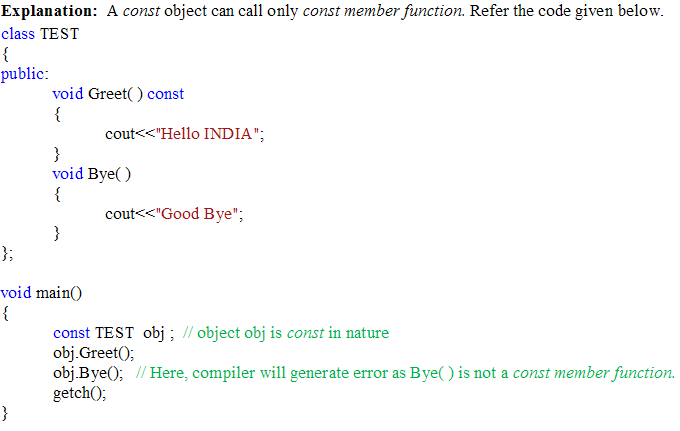# C++ Functions - placement practice test

## C++ Functions - placement practice test

1. Default return type of functions in CPP is

a. void
b. long
c. char
d. int

2. By default, if a function with minimum lines of code is declared and defined inside the class becomes Inline function.

a. True
b. False

3. If a program uses Inline Function, then the function is expanded inline at ___________

a. Compile time
b. Run time
c. Both a and b
d. None of these

4. Inline functions may not work ______

1. If function contain static variables
2. If function contain global and register variables
3. If function returning value consists looping construct(i.e. for, while)
4. If inline functions are recursive
5. If function contains const value

a. Only 1,4,5
b. Only 2,3,5
c. Only 1,3,4
d. All of these

5. Default values for a function are specified when____

a. function is defined
b. function is declared
c. Both a and b
d. None of these

6. Default values for a function are need to be specified from left to right only.

a. True
b. False

Explanation: Default values need to be specified from Right to Left order.
Example:
void calculate(int amt, int years, float rate=7.8); //valid
void calculate(int amt, int years=5, float rate=7.8); //valid
void calculate(int amt=21000, int years, float rate=7.8); //Invalid
Third statement is invalid as we skipped second parameter of the function. Rule says that default values should be set from Right to Left order only. We cannot provide a default value to

specific parameter in the middle of an parameter list.

7. Assigning one or more function body to the same name is called_______________

a. Function Overriding
c. Both a and b
d. None

8. Function overloading can also be achieved if two or more functions differ only in their return types.

a. True
b. False

Explanation: Two functions differing only in their return types cannot be overloaded and hence function overloading can’t be achieved. Try this code and you will get an error.
Example:

9. If an argument to a function is declared as const, then

a. function can modify the argument
b. Function can’t modify the argument
c. const argment to a function is not possible
d. None of these

ANSWER: b. Function can’t modify the argument

10. In any ways, Non-member function cannot have access to the private data of the class.

a. True
b. False

Explanation: Non-member functions can also access private data of the class provided that non-member function should be a friend function of the class.

11. A function can be declared as friend maximum only in two classes.

a. True
b. False

Explanation: A function can be declared as friend in any number of classes.

12. A friend function does not have this pointer associated with it.

a. True
b. False

Explanation: this pointer is passed implicitly as an argument to a function when it is called with object. A friend function is not a member function of a class. And therefore it cannot be

called with objects of the class. And hence, this pointer is not associated with a friend function.

13. Which of the followings is/are not false about friend function ?

1. It can be called / invoked with class object
2. It has objects as arguments
3. It can have built-in types as arguments
4. It must declared only in public part of a class
5. It does not have this pointer as an argument

a. Only 2,4
b. Only 1,2,5
c. Only 2,3,5
d. All of these

14. Can member functions of one class be friend functions of another class?

a. Yes
b. No

15. const member function does not allow to modify/alter value of any data member of the class.

a. True
b. False

16. Can we alter/modify the values of data members of a class inside const member function?

a. Yes
b. No.

Explanation: In a const member function, we can alter the values of only those data members of the class which are declared as mutable inside the class.

17. A const object of a class can call non-const member function of the class.

a. True
b. False